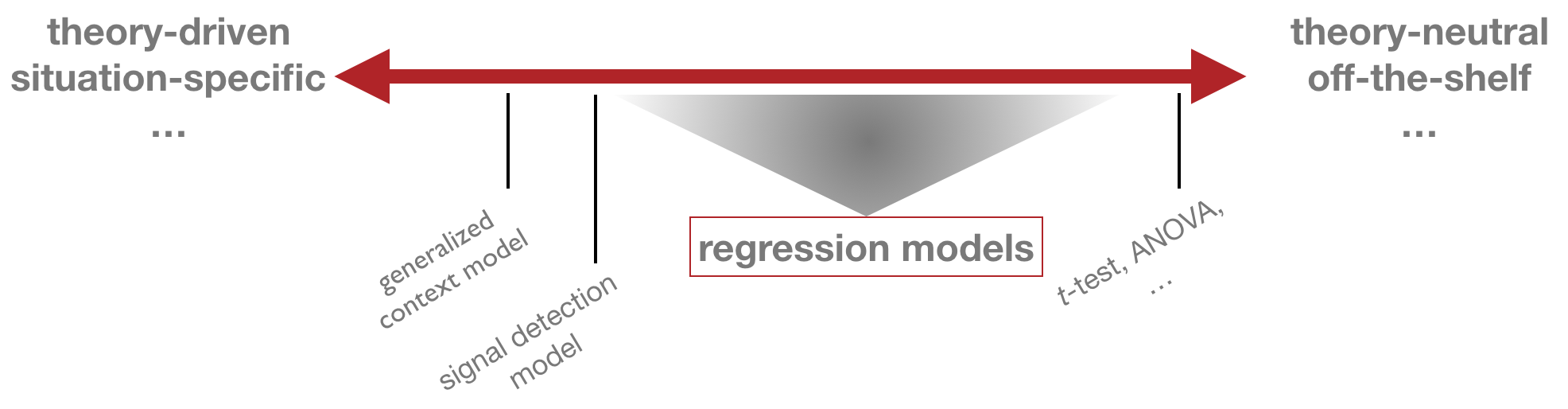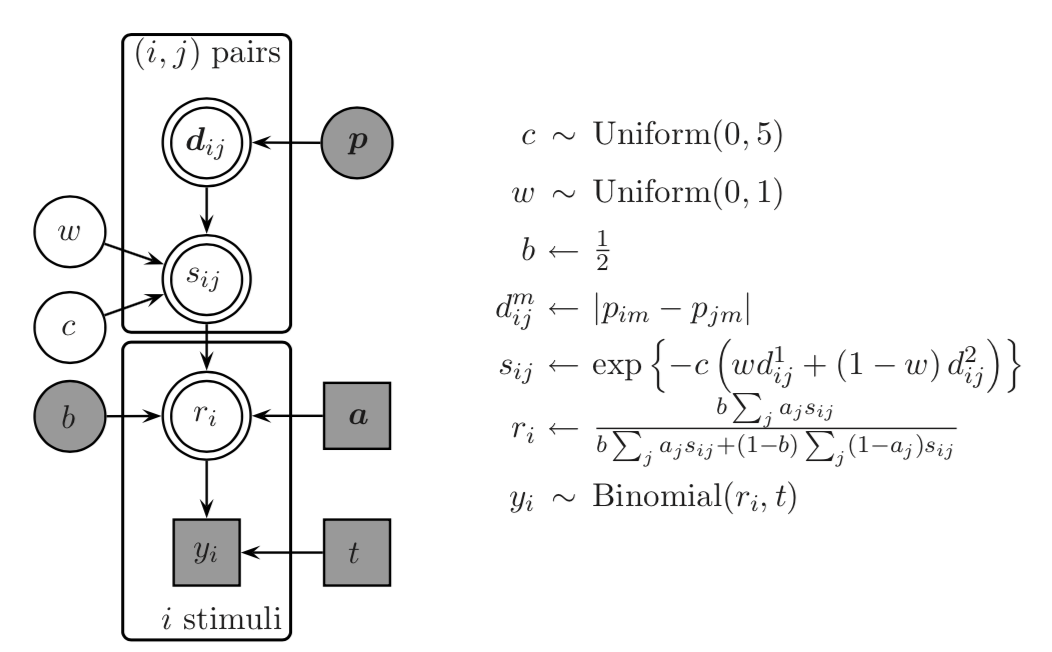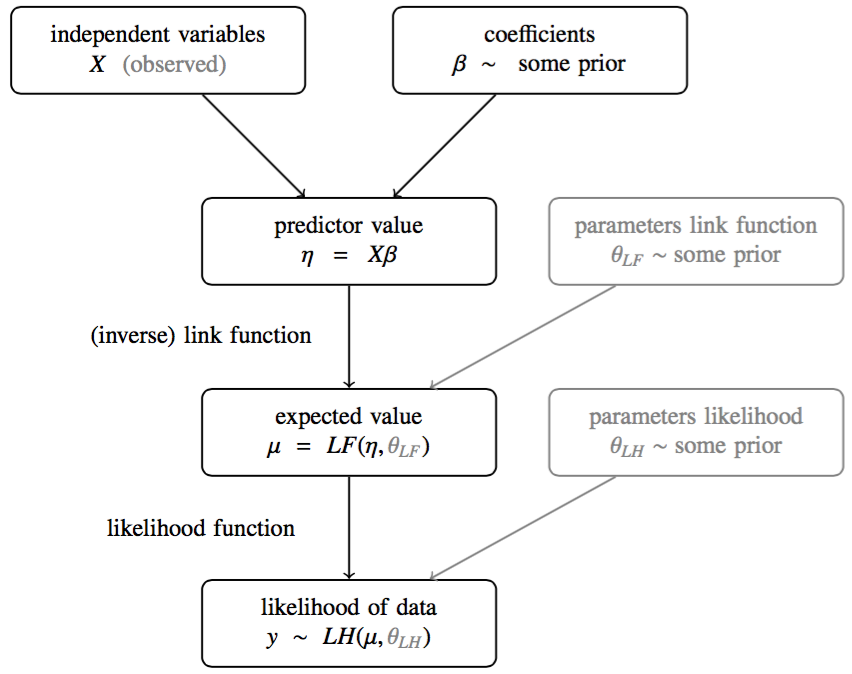$\definecolor{firebrick}{RGB}{178,34,34} \newcommand{\red}{{\color{firebrick}{#1}}}$ $\definecolor{mygray}{RGB}{178,34,34} \newcommand{\mygray}{{\color{mygray}{#1}}}$ $\newcommand{\set}{\{#1\}}$ $\newcommand{\tuple}{\langle#1\rangle}$ $\newcommand{\States}{{T}}$ $\newcommand{\state}{{t}}$ $\newcommand{\pow}{{\mathcal{P}(#1)}}$

## overview

• generalized linear model (GLM)
• types of variables
• metric, nominal, ordinal, count
• linear model
• ordinary least squares regresssion
• maximum likelihood regression
• Bayesian approaches
• generalization to simplest $$t$$-test scenario

## probabilistic models

standard notion

model = likelihood function $$P(D \mid \theta)$$

Bayesian

model = likelihood $$P(D \mid \theta)$$ + prior $$P(\theta)$$

approaches to modeling## Generalized Context Model ::: Model## generalized linear model

terminology

• $$y$$ predicted variable, data, observation, …
• $$X$$ predictor variables for $$y$$, explanatory variables, …

blueprint of a GLM

\begin{align*} \eta & = \text{linear_combination}(X) \\ \mu & = \text{link_fun}( \ \eta, \theta_{\text{LF}} \ ) \\ y & \sim \text{lh_fun}( \ \mu, \ \theta_{\text{LH}} \ ) \end{align*}## types of variables

type examples
metric speed of a car, reading time, average time spent cooking p.d., …
binary coin flip, truth-value judgement, experience with R, …
nominal gender, political party, favorite philosopher, …
ordinal level of education, rating scale judgement, …
count number of cars passing under bridge in 1h, …

## muder rate data set

murder_data = readr::read_csv('data/02_murder_rates.csv') %>%
rename(murder_rate = annual_murder_rate_per_million_inhabitants,
low_income = percentage_low_income,
unemployment = percentage_unemployment) %>%
select(murder_rate, low_income, unemployment,population)
murder_data %>% head
## # A tibble: 6 x 4
##   murder_rate low_income unemployment population
##         <dbl>      <dbl>        <dbl>      <int>
## 1       11.2        16.5         6.20     587000
## 2       13.4        20.5         6.40     643000
## 3       40.7        26.3         9.30     635000
## 4        5.30       16.5         5.30     692000
## 5       24.8        19.2         7.30    1248000
## 6       12.7        16.5         5.90     643000

## visualize data

GGally::ggpairs(murder_data,
title = "Murder rate data")